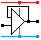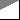Home

rOm
Quest
Glossary

Random
Page
Search
Site
Lush
Sim
Class
Subject
Images

Help
FAQ
Sign
Up
Log
In
 GCSE     Resistors     BS1852     Colour Codes     E24     LDR     >Parallel<     PIVR Calculations     Potentiometer     Puzzles     Series     Thermistor     Timing     Types     Voltage Divider

# Resistors Parallel

Site for Eduqas/WJEC - Go to the AQA site.

GCSE    ResistorsQuestions 0 to 27   -->  View All

Components connected in Parallel are wired together as shown in the diagram.For three resistors ...

Rtotal   =   1 / ( 1 / R1 + 1 / R2 + 1 / R3 )

For two resistors ...

Rtotal   =   1 / ( 1 / R1 + 1 / R2 )   =   R1 R2 / ( R1 + R2 )

You need to be good at fractions or skilled with your calculator!

On a calculator, to get Rtotal press these keys ... 1 / ( 1 / R1 + 1 / R2 ) =

## Combined Series and Parallel

Problems like this need to be solved in stages by breaking the problem into simpler parts.

In the diagram below, add R1 + R2 to work out the series resistors.Then use the parallel resistors formula to work out R3 in parallel with the result of (R1 + R2).

## Another Series and Parallel Calculation

In the next example, work out each of the parallel resistors first and then add the two answers together.(R1 and R2) in parallel added to (R3 and R4) in parallel.

Subject Name     Level     Topic Name     Question Heading     First Name Last Name Class ID     User ID

 CloseEscapeX Q: qNum of last_q     Q ID: Question ID         Score: num correct/num attempts         Date Done

Question Text

image url

Help Text
Debug

• You can attempt a question as many times as you like.
• If you are logged in, your first attempt, each day, is logged.
• To improve your scores, come back on future days, log in and re-do the questions that caused you problems.
• In the grade book, you can delete your answers for a topic before re-doing the questions. Avoid deleting unless you intend re-doing the questions very soon.